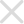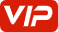## 2020风神奕炫维修手册2• 1.车型介绍
• 车型介绍
• 主要性能参数
• 主要系统参数
• 产品配置表
• 整车技术参数
• 新功能介绍
• 标识牌位置
• 油液规格及容量
• 识别信息
• 车型分类说明
• 车型配置差异
• 车辆外形尺寸
• 10.车身装备
• 10.2玻璃、车窗系统和后视镜
• 准备工作
• 初始化
• 前挡风玻璃
• 后三角玻璃
• 后视镜
• 后风窗玻璃
• 故障诊断
• 注意事项
• 玻璃升降器
• 车门玻璃
• 10.3天窗
• 准备工作
• 天窗
• 故障诊断
• 注意事项
• 10.5仪表板
• 仪表板
• 仪表板分解
• 准备工作
• 副仪表板
• 手套箱
• 故障诊断
• 10.6座椅pdf
• 准备工作
• 前排座椅
• 后排座椅
• 注意事项
• 11.车身检查与结构
• 11.1.车身检查pdf
• 发动机舱测量
• 后车身测量
• 车身前部及侧围测量
• 车身底部测量
• 11.2.车身结构
• 中支柱
• 侧围外板总成
• 前围上挡板带横梁总成
• 前围挡板带挡板中横梁总成
• 前支柱
• 前纵梁总成
• 前翼子板里板加强板总成
• 前轮罩总成
• 前门槛
• 后围板总成
• 后地板中横梁带支架总成
• 后承载式地板
• 后支柱
• 后箱隔板总成
• 后纵梁总成
• 后组合灯座板总成
• 概述
• 水箱立柱焊接总成
• 注意事项
• 焊接基础知识
• 顶棚
• 12.空调系统
• 冷冻油
• 准备工作
• 制冷剂的回收抽真空充注查漏
• 故障诊断
• 注意事项
• 温度传感器
• 空调伺服电机
• 空调冷凝器
• 空调出风口
• 空调压力开关
• 空调压缩机
• 空调控制单元
• 空调控制面板
• 空调暖风水箱
• 空调管路组件
• 空调线束
• 空调膨胀阀
• 空调蒸发器
• 系统概述
• 维修数据及规格参数
• 阳光传感器
• 鼓风机
• 13.电器系统
• 13.1.起动和充电系统-赵迪
• 准备工作
• 发电机
• 系统概述
• 蓄电池
• 起动机
• 13.10.CAN网
• 故障诊断
• 网关控制器
• 13.2.智能进入系统
• 一键启动开关
• 售后匹配学习
• 故障诊断
• 智能进入（PEPS）
• 注意事项
• 系统概述
• 车身控制器（IBCM）
• 13.3.照明
• 光线雨量传感器
• 准备工作
• 前照灯
• 前雾灯
• 危险报警开关
• 后照灯
• 后视镜转向灯
• 故障诊断
• 维修数据及规格参数
• 行李箱灯
• 车灯位置
• 13.5.雨刮
• 光线雨量传感器
• 前雨刮传动机构
• 喇叭
• 故障诊断
• 注意事项
• 清洗器
• 雨刮组合开关
• 13.5.驾驶员信息系统-赵迪
• 故障诊断
• 注意事项
• 系统概述
• 组合仪表
• 13.6.车身控制
• 多功能开关
• 故障诊断
• 注意事项
• 系统概述
• 车身控制器（IBCM）
• T-BOX控制器
• 故障诊断
• 13.9.影音系统
• 准备工作
• 多媒体影音系统
• 扬声器
• 收音机天线
• 故障诊断
• 泊车辅助系统
• 电器接口
• 紧急制动辅助（AEB）雷达传感器
• 自适应巡航摄像头
• 14.故障诊断
• ACU气囊系统
• AC空调系统
• AVM360环视控制单元
• BCM车身控制单元
• CAMERA车道偏离预警摄像头
• EPS电子助力转向系统
• ESC车身控制单元
• ESM电子换档器
• GBCP档电机
• GW网关系统
• IC组合仪表
• MFS中控台开关
• MP5影音系统
• SCC方向盘快捷键
• T-BOX系统
• 英文缩写翻译
• 诊断工作流程
• 15.附录（关于本维修手册使用）
• 如何使用本手册
• 注意事项
• 索引
• 2.新车准备-D53
• 东风风神新车准备检查记录表
• 新车准备
• 前期准备
• 操作规程
• 3.保养
• 东风风神车辆保养记录表
• 保养里程
• 关键力矩点
• 准备工作
• 发动机舱保养
• 定期保养
• 日常保养
• 注意事项
• 维修保养最终检查
• 维修数据和规格
• 车身和底盘保养
• 4.发动机
• 4.1.发动机结构-A15T
• 准备工作
• 双质量飞轮总成
• 发动机-变速器总成
• 发动机弹性支架
• 发动机线束
• 变速器分离
• 注意事项
• 4.2.发动机润滑-A15T
• 准备工作
• 发动机机油
• 故障诊断
• 油底壳
• 注意事项
• 系统概述
• 4.2.发动机润滑系统.-C10TD
• 准备工作
• 发动机机油
• 密封件
• 故障诊断
• 油底壳
• 注意事项
• 系统概述
• 4.3.发动机冷却系统-A15T
• 冷却水泵
• 冷却液
• 冷却风扇
• 准备工作
• 出水室
• 故障诊断
• 散热器
• 散热器进、出水管
• 暖风水管
• 暖风水管（金属管）
• 机油冷却器进、出水管
• 注意事项
• 涡轮增压器进、出水管
• 系统概述
• 维修数据及规格参数
• 膨胀水箱
• 膨胀水箱通气管
• 节温器
• 4.3.发动机冷却系统-C10TD
• 冷却模块
• 冷却水泵
• 冷却液
• 冷却管路
• 冷却风扇
• 准备工作
• 故障诊断
• 散热器
• 注意事项
• 系统概述
• 维修数据及规格参数
• 膨胀水箱
• 膨胀水箱出水管
• 膨胀水箱通气管
• 节温器
• 4.4.发动机控制-A15T
• VVT阀
• 凸轮轴位置传感器
• 加速踏板
• 发动机冷却液温度传感器
• 发动机控制单元
• 发动机爆震传感器
• 售后匹配学习
• 增压压力与温度传感器
• 机油压力传感器
• 氧传感器
• 注意事项
• 碳罐控制阀
• 系统概述
• 转速传感器
• 进气压力传感器
• 进气温度传感器
• 4.5.发动机燃油系统-C10TDpdf
• 准备工作
• 故障诊断
• 注意事项
• 燃油喷射机构
• 燃油泵及燃油箱
• 燃油管路及滤清器
• 碳罐及燃油蒸气管
• 系统概述
• 高压油泵
• 4.5.燃油系统-A15T
• 准备工作
• 故障诊断
• 注意事项
• 燃油喷射机构
• 燃油泵及燃油箱
• 燃油管路及滤清器
• 碳罐及燃油蒸气管
• 系统概述
• 4.6.进排气系统-A15T
• 中冷器
• 准备工作
• 前进气口带进气管总成
• 增压器电子泄压阀
• 排气管
• 故障诊断
• 注意事项
• 涡轮增压器
• 电子节气门体
• 空气滤清器
• 系统概述
• 维修规格及技术参数
• 进气歧管
• 4.6.进排气系统-C10TD
• GPF积碳处理
• 三元催化器
• 中冷器
• 准备工作
• 压差传感器
• 增压器电子泄压阀
• 排气温度传感器
• 排气管
• 故障诊断
• 注意事项
• 涡轮增压器
• 电子节气门体
• 空气滤清器
• 系统概述
• 维修规格及技术参数
• 进气口
• 进气歧管
• 5.变速器与离合器
• 5.1.离合器
• 准备工作
• 故障诊断
• 注意事项
• 离合器排气
• 离合器检查
• 离合器踏板机构
• 系统概述
• 维修数据及规格
• 5.2.手动变速器
• 准备工作
• 变速器总成
• 变速器控制
• 变速器油封
• 变速器油液
• 故障诊断
• 注意事项
• 系统概述
• 维修数据及规格
• 5.3.双离合变速器
• 传感器
• 准备工作
• 变速器总成
• 变速器油液
• 变速器电控单元
• 变速器线束
• 换档控制总成
• 故障诊断
• 油封
• 注意事项
• 电机
• 离合器泵
• 系统概述
• 维修数据及规格
• 自学习
• 6.车桥与悬架
• 6.1.前桥和前悬架
• 三角臂
• 准备工作
• 前减震器
• 前桥与前悬架
• 前横向稳定杆
• 半轴
• 发动机托架
• 故障诊断
• 注意事项
• 系统概述
• 维修数据及规格
• 转向节
• 6.2.后桥与后悬架
• 准备工作
• 后减震器
• 后托架
• 后轮毂及轴承
• 故障诊断
• 注意事项
• 系统概述
• 6.3.车轮
• 准备工作
• 四轮定位
• 故障诊断
• 系统概述
• 车轮
• 7.制动
• 7.1.制动系统-赵迪
• 准备工作
• 制动液
• 前制动系统组件
• 后制动系统组件
• 故障诊断
• 注意事项
• 维修数据与规格参数
• 7.2.驻车制动系统
• 准备工作
• 注意事项
• 驻车制动组件
• 7.3.制动控制系统-赵迪
• ESC液压单元
• 准备工作
• 故障诊断
• 注意事项
• 系统概述
• 轮速传感器
• 8.转向
• EPS配置信息
• 准备工作
• 故障诊断
• 方向盘
• 检查与调整
• 注意事项
• 维修数据与规格
• 转向器总成
• 转向柱总成
• 转向柱锁总成
• 转角自学习
• 9.约束系统
• 9.1.座椅安全带pdf
• 前排座椅安全带
• 后排座椅安全带
• 系统概述
• 9.2辅助约束系统pdf
• 准备工作
• 前乘客气囊
• 前座椅气囊
• 故障诊断
• 气囊电控单元
• 注意事项
• 碰撞传感器
• 配置写入
• 驾驶员气囊